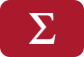## Error message

• Deprecated function: Methods with the same name as their class will not be constructors in a future version of PHP; ctools_context has a deprecated constructor in require_once() (line 127 of /home/tusa/public_html/sites/all/modules/ctools/ctools.module).
• Deprecated function: Methods with the same name as their class will not be constructors in a future version of PHP; ctools_context_required has a deprecated constructor in require_once() (line 127 of /home/tusa/public_html/sites/all/modules/ctools/ctools.module).
• Deprecated function: Methods with the same name as their class will not be constructors in a future version of PHP; ctools_context_optional has a deprecated constructor in require_once() (line 127 of /home/tusa/public_html/sites/all/modules/ctools/ctools.module).
• Deprecated function: Methods with the same name as their class will not be constructors in a future version of PHP; panels_cache_object has a deprecated constructor in require_once() (line 127 of /home/tusa/public_html/sites/all/modules/ctools/ctools.module).

# Free Calculus Worksheets100% Free Calculus Worksheets, Printables, and Activities.

Our downloadable and printable Calculus Worksheets cover a variety of calculus topics including limits, derivatives, integrals, and more. All of our worksheets are free for use by teachers, students, homeschool parents teaching calculus, or anyone using them in an educational setting.

Calculus Teachers

Your time is valuable. Our worksheets are great for both classroom assignments and homework.

Students

It's hard to master calculus without practice. Our worksheets are perfect for students looking for a little extra practice before the big exam.  All of our calculus printables contain an answer key so you can immediately check your work.

Homeschool Parents

Our free calculus worksheets are a great supplement to any home school curriculum.

Below you can find our collection of worksheets for calculus.

In this 100% free calculus worksheet, students must find Taylor and Maclaurin Polynomials of a stated degree with the Langrange form of the remainder.
Worksheet (Calculus)
Problems: 10
In this free calculus worksheet, students must find limits of problems where the limit is approaching positive infinity or negative infinity.
Worksheet (Calculus)
Problems: 10
In this free printable calculus worksheet, students must use rules of differentiation to find the derivative of polynomial expressions.
Worksheet (Calculus)
Problems: 10
In this 100% free calculus worksheet, students are asked to find the value of multivariable limits (limits in 2 variables). First students should determine if limit exists or does not exist. Then...
Worksheet (Calculus)
Problems: 10
In this printable free calculus worksheet, students must find values of limits in two variables. Students should first determine if the limit exists and if they do give the value of the limit.
Worksheet (Calculus)
Problems: 10
This printable calculus worksheet contains problems on calculus limits. Students must use their knowledge of limits to solve problems.
Worksheet (Calculus)
Problems: 10
This free calculus worksheet contains problems on antidifferentiation and indefinite integrals.
Worksheet (Calculus)
Problems: 10
This free calculus worksheet contains problems on hyperbolic functions. Students must find the value of hyperbolic functions and also find derivatives and integrals.
Worksheet (Calculus)